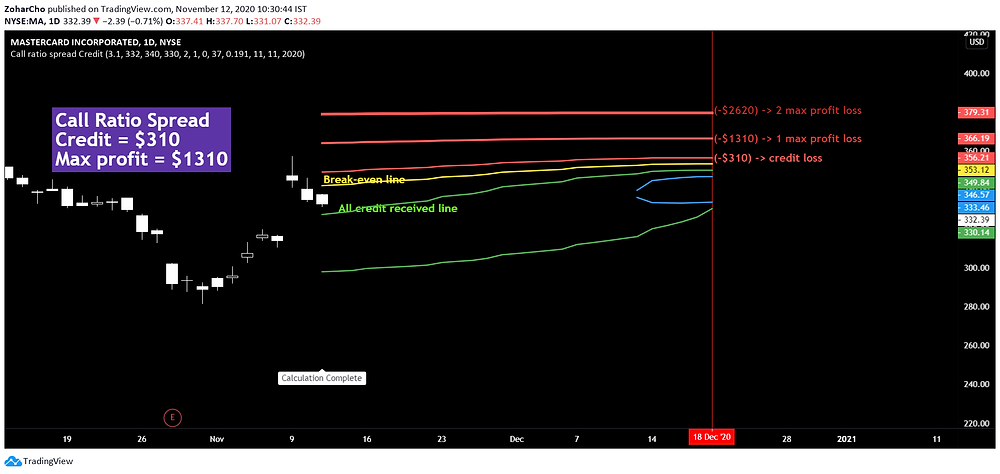Search
• support10070

# Call Ratio Spread CreditA ratio call spread is a neutral strategy in which we buy several calls at a lower strike and sells more calls at a higher strike. In a ratio call spread with credit, there is no downside risk. The ratio spread that we see on the chart has a ratio of 2:1.

We can see from the chart the non-linear behavior of options.

Inputs: MA (Mastercard)

Credit received -> 3.1 (\$310 for one position)

Stock price -> 332

Upper strike -> 340 , 2 calls sold

Lower strike -> 330 , 1 call bought

Days to expire -> 37

Implied Volatility -> 0.291 (29.1%)

Date -> 11/11/2020

The credit received is \$310, the maximum profit is \$1310 with less than 1% probability, the maximum loss is theoretically unlimited.

In this example, one call was bought at 330 strike price for 14.2 and two calls were sold at 340 strike price for 8.65 each, in total 17.3.

The credit = 17.3-14.2 = 3.1

If at expiration the stock price will be below the lower strike (330), all of the options will be worthless and all the credit will be received.

The maximum profit at expiration for a ratio spread occurs if the stock is exactly at the striking price of the sold options. The reason is that the call that was bought has some profit (stock price above strike price) and the sold options are worthless.

Maximum profit = The spread (340-330=10) + Credit received (17.3) – Debit paid (14.2) = 13.1 => \$1310 (mulitpling by 100 shers per option contract)

The risk in this position is to the upside. The calculation for the break-even at expiration.

Break-even point = Upper strike price + the points of max profit = 340+13.1=353.1

This strategy has a high probability in general and even more so when used correctly.

The example that has been used could profit the most in the blue zone, where the profit is greater than 50% of the maximum profit, but it will take 34 days out of 37 to reach there.

How implied volatility affect this position?

In a ratio spread, there are more options sold than bought, in the previous posts we saw that volatility increase is harming sold options and benefits bought options, this example is no different.

10% increase in implied volatility, the lines are now in a worse location compare to the original position.10% decrease in implied volatility, the lines are now in a better location compare to the original position. The position can now reach the 50% max profit zone in 30 days.The next post will be on ratio spread debit, that looks different from the ratio spread credit, the solution to the partial differential equations of the Black-Scholes model can be seen.

5 views0 comments

See All